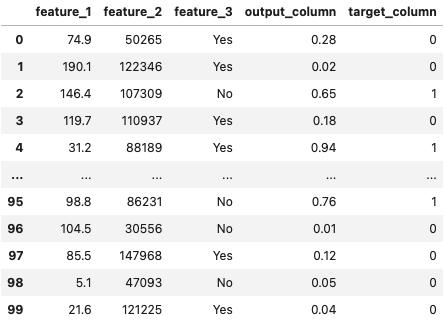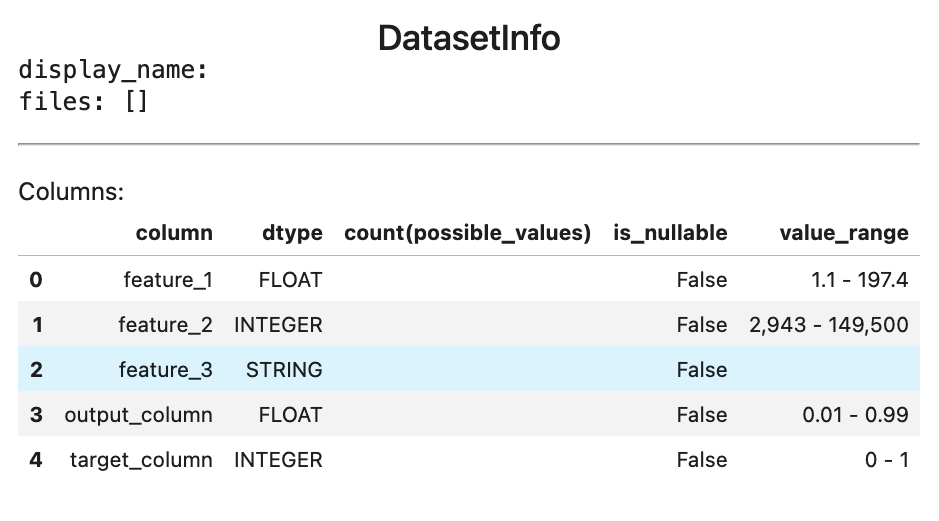# Customizing Your Dataset Schema

It's common to want to modify your `fdl.DatasetInfo` object in the case where something was inferred incorrectly by `fdl.DatasetInfo.from_dataframe`.

Let's walk through an example of how to do this.

Suppose you've loaded in a dataset as a pandas DataFrame.

``````import pandas as pd

``````

Below is an example of what is displayed upon inspection.Suppose you create a `fdl.DatasetInfo` object by inferring the details from this DataFrame.

``````dataset_info = fdl.DatasetInfo.from_dataframe(df)
``````

Below is an example of what is displayed upon inspection.But upon inspection, you notice a few things are wrong.

1. The value range of `output_column` is set to `[0.01, 0.99]`, when it should really be `[0.0, 1.0]`.
2. There are no possible values set for `feature_3`.
3. The data type of `feature_3` is set to `fdl.DataType.STRING`, when it should really be `fdl.DataType.CATEGORY`.

Let's see how we can address these issues.

# Modifying a column’s value range

Let's say we want to modify the range of `output_column` in the above `fdl.DatasetInfo` object to be `[0.0, 1.0]`.

You can do this by setting the `value_range_min` and `value_range_max` of the `output_column` column.

``````dataset_info['output_column'].value_range_min = 0.0
dataset_info['output_column'].value_range_max = 1.0
``````

# Modifying a column’s possible values

Let's say we want to modify the possible values of `feature_3` to be `['Yes', 'No']`.

You can do this by setting the `possible_values` of the `feature_3` column.

``````dataset_info['feature_3'].possible_values = ['Yes', 'No']
``````

# Modifying a column’s data type

Let's say we want to modify the data type of `feature_3` to be `fdl.DataType.CATEGORY`.

You can do this by setting the `data_type` of the `feature_3` column.

``````dataset_info['feature_3'].data_type = fdl.DataType.CATEGORY
``````